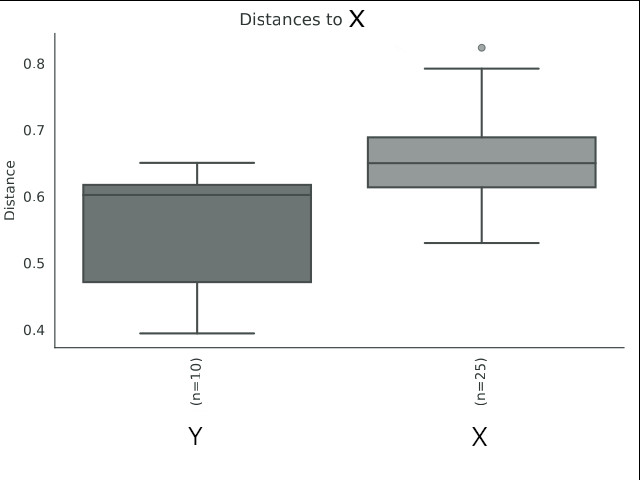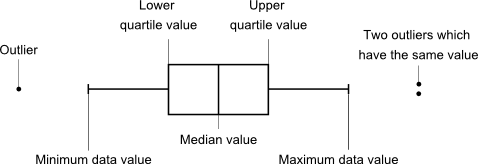# Bray Curtis Distance labels

Dear team,

I wanted to ask you about the type of plot and labels produced by the bray curtis distance matrix plot (qiime2 pipeline) and the most accurate way to report the output. The plot auto outputs as index, which means it's a bray curtis index and not a dissimilarity plot? And I'm presuming the labels n = x indicates number of species in each site. How would qiime2 developers interpret this plot? I want to make sure this is as accurate as possible and that site X is indeed sig. dissimilar to site Y.
Details:
|Group 1|Group 2|Sample size|Permutations|pseudo-F|p-value|q-value|
|Y|X|10|999|3.696546533|0.014|0.014|1 Like

Ah, I think I have found the point of confusion. In this case, the index is a dissimilarity.

This is a good question, because some beta diversity indexes are true distances, while the alpha diversity indexes are all sorts of things (counts, coefficients, probabilities, etc)

3 Likes

Thank you very much for clarifying this, I wasn’t quite sure which calculation was used.

Ah, also, N is the number of pairwise comparisons made.

So Y (n=10) means there were ten pairs of samples compared between Y and X.

For example, 2 samples in y and 5 samples in x, thus 2*5=10 dissimilarities with a median of 0.6.

1 Like

Ah, of course it means comparisons, I've been looking at too many plots! Thank you very much for your help. I wanted to also double check if the dot there is an outlier? Can one trace back to what feature/taxa that outlier represents?

1 Like

This can depend on the plotting library, so let's see if someone can point us to the official docs on what exactly the boxes, and whiskers, and dots mean.

The input to these graphs are dissimilarities between pairs of samples. So a high outlier would be a pair of samples that are super dissimilar. You could find out what those two samples are.

The beta diversity box plots are for a comparison of means between groups. You can also compare groups using a PCoA plot. Have you done that? What to post a PCoA plot colored by group so we can take a look?Thank you very much for your response. My understanding is that the boxes in the plot represent the interquartile ranges, the horizontal lines give the position of the medians, the vertical bars indicate the range. The dots indicate outliers.I have done this yes and did see where the possible outliers indicated were. I think I shall need to dig deeper into the output files to see exactly which dot belongs to which sample. Thank you again for your help, it is greatly appreciated'Minimum data value' so the minimum is not the lowest value observed?
'Two outliers are stacked' Sometimes! Or they can be plotted on top of each other and be a single point.This is the danger of 'Googleing it' or asking chatGPT. It's all assumptions.

If you want to know for sure, you can check what the plugin is REALLY doing.Here's the code for distance box plots: q2-diversity/_visualizer.py at master · qiime2/q2-diversity · GitHub
This uses a seaborn box plot.

Colin, I agree re: chatGPT. My understanding comes from undergraduate training in the area: